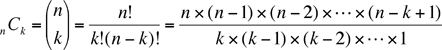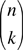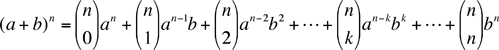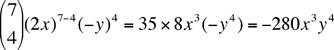Name: April Who is asking: Student Level of the question: Secondary Question: What are the 3rd and 4th terms of this sequence: (2x-y)7? I'm having an issue with this...is there any easier way to get it without completely factoring the whoooole thing out? Hi April, There certainly is a better way then expanding the entire expression, it is to use the binomial theorem. You need to use the binomial coefficients which you may have seen before. For integers n and k, with k ≤ n, the binomial coefficients are given by.I wrote the notation nCk since some high school texts use this notation but I am going to usesince this is the notation mathematicians use. Using this notation the binomial theorem states.For your example you have n = 7, a = 2x and b = -y. Hence, for example the fifth term isPenny